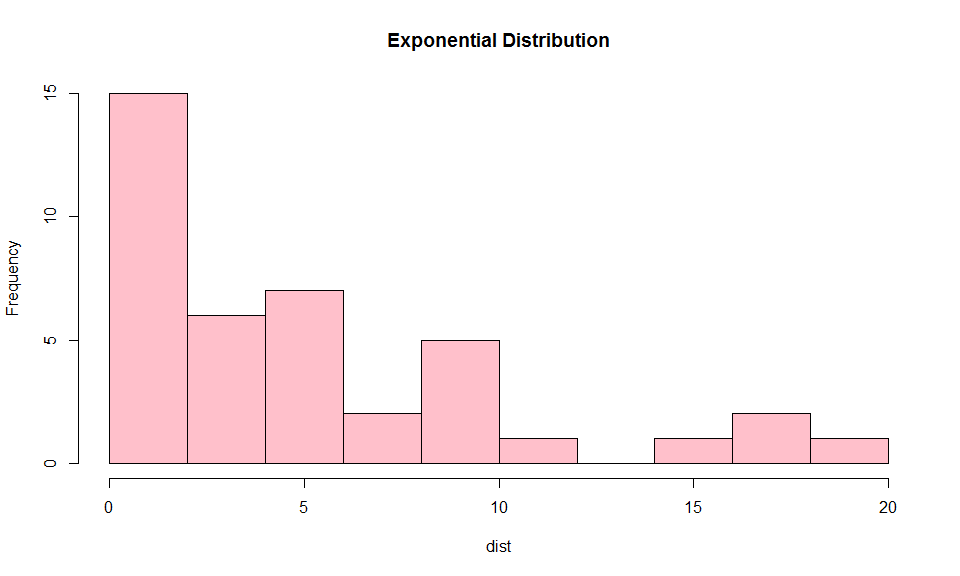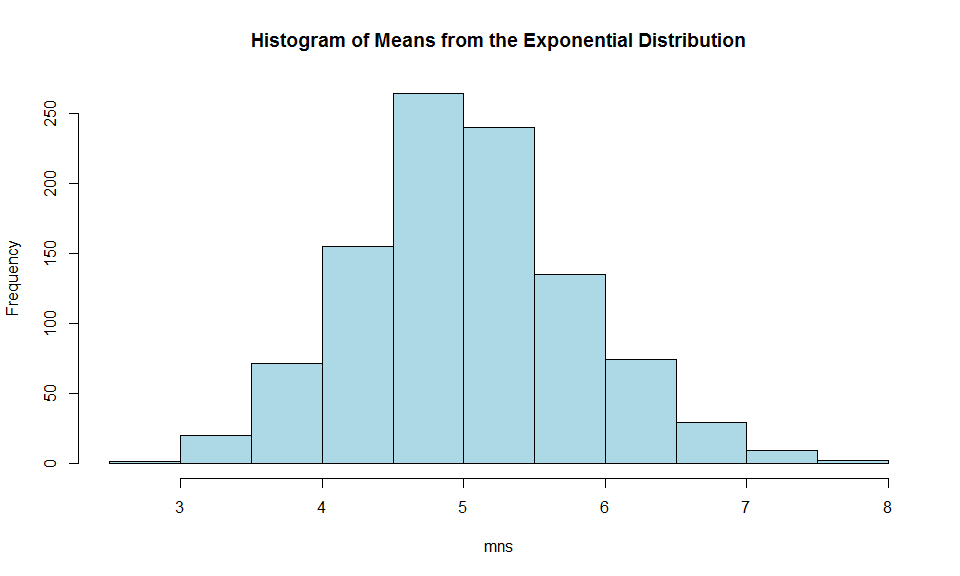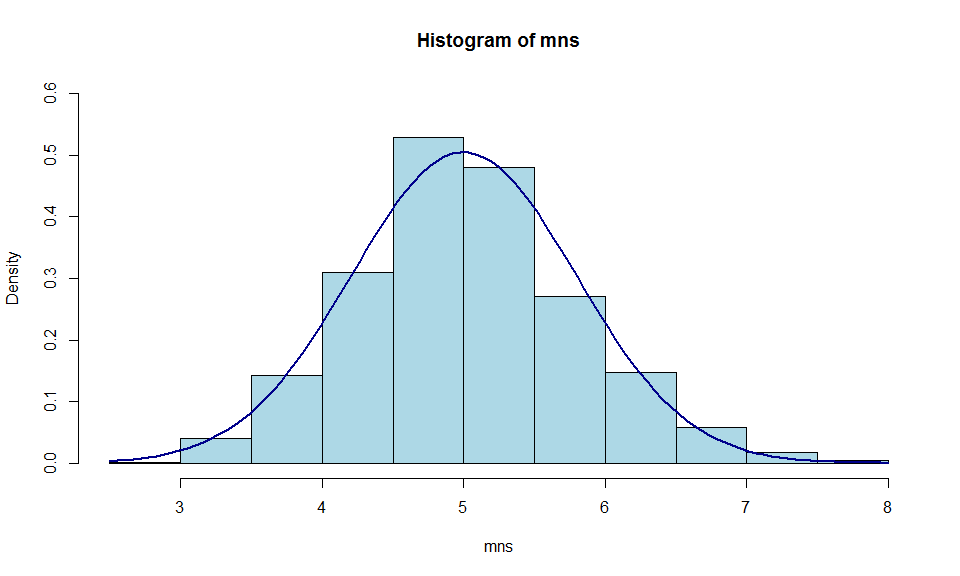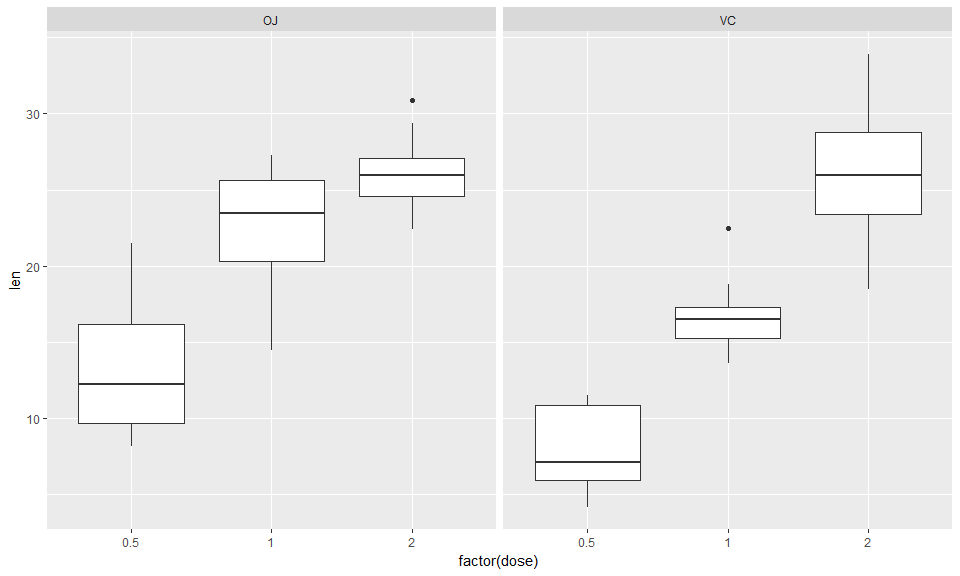library(ggplot2)

## Part 1: Investigate the Exponential Distribution

### Overview

The purpose of this exercise is to investigate the exponential distribution in R and compare it with the Central Limit Theorem.

To begin, the shape of the exponential distribution on random samples generated for 40 observations is shown in the histogram below. You can see that its shape does not look symmetrical (as expected).

# Create a sample of 40 random observations from the exponential distribution
dist <- rexp(40,.2)

# Record the mean, variance, and standard deviation from this sample.
distMean <- mean(dist)
distVar <- var(dist)
sdVar <- sd(dist)

# Show the histogram
hist(dist, main="Exponential Distribution", col="pink")Histogram of 40 random observations from the exponential distribution

Statistics of this distribution include,

• mean: 5
• variance: 25.92
• Std. Deviation: 5.09

### Simulations

Now, let’s do this again 1,000 times and record the mean for each iteration.

# Create a variable to hold the mean for each simulated distribution
mns = NULL

# Generates random variables in the exponential distribution within a loop 1,000 times
# Take the means of each distribution and concatenate the mns variable

for (i in 1 : 1000) {
mns = c(mns, mean(rexp(40,.2)))
}

### Mean: Sample VS Theoretical

The mean of a single distribution is the sample or empirical mean (the estimators). In the distribution above the mean was: 5. Each time you generate a distribution, you generate another mean. All these means are themselves randomly distributed variables. As you collect more samples, their distribution means converge on the theoretical mean and take a normally distributed shape. The mean of the means from the simulation is 5.02. The histogram below shows the 1,000 means taken from the simulated distributions. This mean is very close to what we know to be true of the exponential distribution mean $$\frac{1}{\lambda}$$ or $$\frac{1}{.2}=5$$ in this case.

hist(mns,main="Histogram of Means from the Exponential Distribution", col="lightblue")Histogram of 1,000 means of exponential distributions

This illustrates that the means of non-normal distribution samples are normally distributed and cantered on the theoretical, or population mean.

### Variance: Sample VS Theoretical

As with the mean, the sample variance estimates the population variance. In this case, we can calculate the sample variance by using the formula $$\left(\frac{\sigma}{\sqrt{n}} \right)^2$$. We know the standard deviation is $$\frac{1}{\lambda}$$ or $$\frac{1}{.2}=5$$ (the same as the mean for exponential distributions). Which is a variance of $$\left(\frac{5}{\sqrt{40}} \right)^2=$$.625 in this case. The variation between the means in the simulated data should be close and in our example is: 0.611.

### Distribution

The plot below shows the histogram of the means as in the figure above, but this time with a normal curve using the population mean and standard deviation

hist(mns, prob=TRUE, ylim=c(0, .6), col="lightblue")
curve(dnorm(x, mean=5, sd=sqrt(.625)),col="darkblue", lwd=2, add=TRUE)Histogram with Normal Plot

The means are nearly normally distributed and will become closer as more samples are included.

## Part 2: Tooth Growth Analysis

### The Tooth Growth Data

This data is from a study of 60 guinea pigs where each animal was given daily amounts of vitamin C. There were three dosages, .5, 1, 2 mg / day delivered through to methods, orange juice (OJ) and ascorbic acid (VC). The study measures the length of teeth in response to these variables.

data(ToothGrowth) 

This analysis of tooth growth data shows that tooth growth may be influenced by daily vitamin C consumption. The 60 observations are broken down into six combinations of dosage amounts and delivery methods each containing 10 observations as shown in the table below. Note that the data is not grouped, there are 60 observations for 60 different guinea pigs.

table(ToothGrowth[,2:3]) 
##     dose
## supp 0.5  1  2
##   OJ  10 10 10
##   VC  10 10 10

The visual inspection shows that dosage could be an influence on tooth growth. It is less clear if there is a significant impact from the delivery method. The hypothesis test will clarify.

ggplot(ToothGrowth, aes(x=factor(dose), y=len)) + geom_boxplot() + facet_grid(~supp)Tooth Growth Lengths & Factors

### Hypothesis tests

Hypothesis testing will help identify the significant factors to tooth growth. Below are two questions and answers relating to dosage and delivery methods.

Question 1: Is the mean of tooth length the same or different between delivery methods?

First some basic statistics (confidence interval (CI) are at 95%),

OJ: mean = 20.66, sd = 6.61, mean CI = 18.2, 23.13

VC: mean = 16.96, sd = 8.27, mean CI = 13.88, 20.05

The calculations for these value are as follows:

# The center and spread of tooth length by delivery method...
mean(ToothGrowth[ToothGrowth$supp == "OJ",]$len)
sd(ToothGrowth[ToothGrowth$supp == "OJ",]$len)

mean(ToothGrowth[ToothGrowth$supp == "VC",]$len)
sd(ToothGrowth[ToothGrowth$supp == "VC",]$len)

# the 95% confidence interval for tooth length for OJ delivery method
mean(ToothGrowth[ToothGrowth$supp == "OJ",]$len) + c(-1,1) * qt(.975, df=length(ToothGrowth[ToothGrowth$supp == "OJ",]$len)-1) * sd(ToothGrowth[ToothGrowth$supp == "OJ",]$len)/sqrt(length(ToothGrowth[ToothGrowth$supp == "OJ",]$len))

# the 95% confidence interval for tooth length for OJ delivery method
mean(ToothGrowth[ToothGrowth$supp == "VC",]$len) + c(-1,1) * qt(.975, df=length(ToothGrowth[ToothGrowth$supp == "VC",]$len)-1) * sd(ToothGrowth[ToothGrowth$supp == "VC",]$len)/sqrt(length(ToothGrowth[ToothGrowth$supp == "VC",]$len))

The mean for VC is just below the 95% confidence interval for the mean tooth length from OJ Conversely, the mean for OJ is above confidence interval for VC. Although, at a 95% confidence, it is pretty close.

Because it is so close, a hypothesis test will calculate the likelihood that these groups are different.

Use a two-sided Students t-Test,

• Ho: The mean of tooth len for VC is the same as the mean for tooth length of OJ (difference in $$means = 0$$)
• Ha: The mean of tooth len for VC is not the same as the mean for tooth length of OJ (difference in $$means \neq 0$$)
t.test(len ~ supp, alternative = "two.sided", paired = FALSE, var.equal=FALSE, data = ToothGrowth, conf.level = 0.95)
##
##  Welch Two Sample t-test
##
## data:  len by supp
## t = 1.9153, df = 55.309, p-value = 0.06063
## alternative hypothesis: true difference in means is not equal to 0
## 95 percent confidence interval:
##  -0.1710156  7.5710156
## sample estimates:
## mean in group OJ mean in group VC
##         20.66333         16.96333

The confidence interval includes zero suggesting that both groups have the same tooth length. Additionally, a p-value of 6% suggests that there is a better than 5% chance that we would observe this scenario if the null hypothesis is true. Therefore, we fail to reject the null hypothesis and conclude there is not enough evidence to suggest the delivery type is a significant factor in tooth growth.

Question 2: Is there a difference in the amount of tooth growth based on the amount (dosage) of vitamin C?

Because there seems to be no significant difference in delivery method, we will consider dosage without regard to delivery method. We will pair the tooth length at a dosage of .5 with that of 2.

• Ho: .5 tooth length = 2 tooth length
• Ha: .5 tooth length < 2 tooth length
# Isolate data where dose = .5 and 2...
TGLen1 <- ToothGrowth[ToothGrowth$dose == .5,] TGLen2 <- ToothGrowth[ToothGrowth$dose == 2,]

t.test(TGLen1$len, TGLen2$len, paired=FALSE, alternative = "less", conf.level = .95, var.equal = FALSE)
##
##  Welch Two Sample t-test
##
## data:  TGLen1$len and TGLen2$len
## t = -11.799, df = 36.883, p-value = 2.199e-14
## alternative hypothesis: true difference in means is less than 0
## 95 percent confidence interval:
##       -Inf -13.27926
## sample estimates:
## mean of x mean of y
##    10.605    26.100

In this case, we clearly reject the null hypothesis, that the means from the two groups are equal. Dosage is a significant factor to tooth growth.

### Conclusion

We conclude that tooth growth appears to be impacted by the amount of vitamin C consumed daily. The delivery method for vitamin C, however, has no significant bearing. Assumptions are that the sample is a sufficient representation of the population (thus using the t-distribution) and a confidence interval of 95% is prudent.